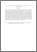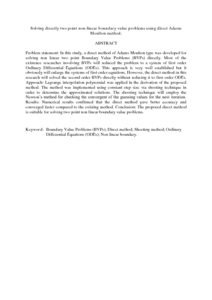# Solving directly two point non-linear boundary value problems using direct Adams Moulton method.

## Citation

Abdul Majid, Zanariah and Phang, Pei See and Suleiman, Mohamed (2011) Solving directly two point non-linear boundary value problems using direct Adams Moulton method. Journal of Mathematics and Statistics, 7 (2). pp. 124-128. ISSN 1549-3644

## Abstract

Problem statement: In this study, a direct method of Adams Moulton type was developed for solving non linear two point Boundary Value Problems (BVPs) directly. Most of the existence researches involving BVPs will reduced the problem to a system of first order Ordinary Differential Equations (ODEs). This approach is very well established but it obviously will enlarge the systems of first order equations. However, the direct method in this research will solved the second order BVPs directly without reducing it to first order ODEs. Approach: Lagrange interpolation polynomial was applied in the derivation of the proposed method. The method was implemented using constant step size via shooting technique in order to determine the approximated solutions. The shooting technique will employ the Newton’s method for checking the convergent of the guessing values for the next iteration. Results: Numerical results confirmed that the direct method gave better accuracy and converged faster compared to the existing method. Conclusion: The proposed direct method is suitable for solving two point non linear boundary value problems.Preview
PDF (Abstract)
Solving directly two point non.pdfView Item